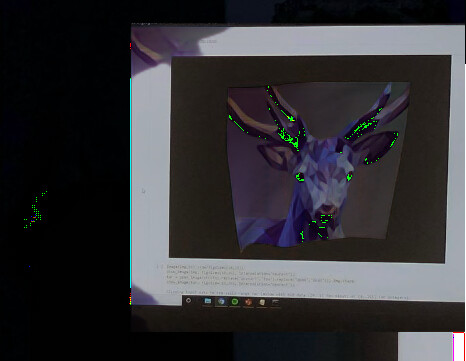# Problems saving inferred image Tensor using cv2

I have used my saved learner model to infer some images. The code I’m using is:

`p,img_hr,b = learn.predict(img)`

`Image(img_hr).show(figsize=(18,18))`

This gives me my desired image but I don’t know how to write the image to a file. I know I can imwrite the image with cv2 but I get a strange result when trying to use cv2 to show the image.

`img_hr=torchvision.transforms.ToPILImage()(img_hr)`
`img_hr= numpy.array(img_test)`
`cv2.imshow(img_hr)`

This gives me an almost correct image but with bright coloured pixels (mostly green but also red blue) over the image which shouldn’t be thereI’m guessing there is some error in my method for converting the tensor to a numpy image (the bright pixels suggest to me there’s an issue with clipping but I don’t really understand why that’s happening). If anyone can see what’s going wrong I’d appreciate the help. Or if anyone can suggest a better method of saving the tensor as an image.

Don’t know what went wrong but this works:

``````p,img_hr,b = learn.predict(img)
im=image2np(Image(img_hr).data).astype(np.float32)
im=np.clip(im, 0,1)*255
cv2_imshow(im)``````
1 Like